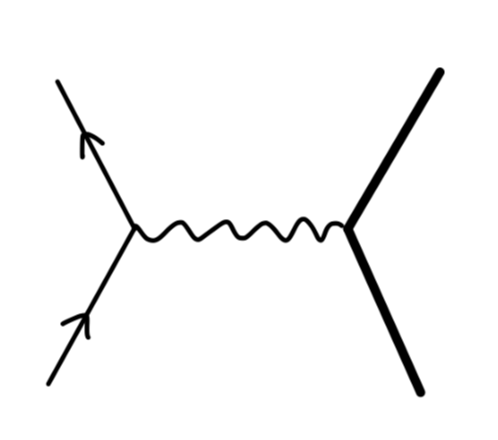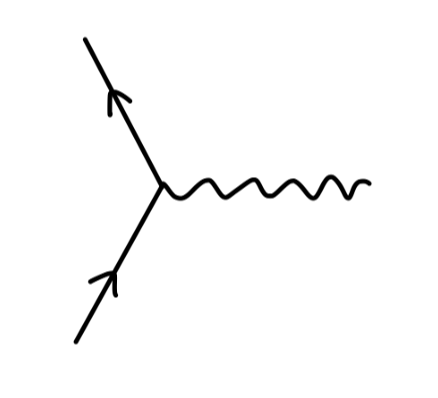$-ie\gamma^{\mu}$

• external leg correction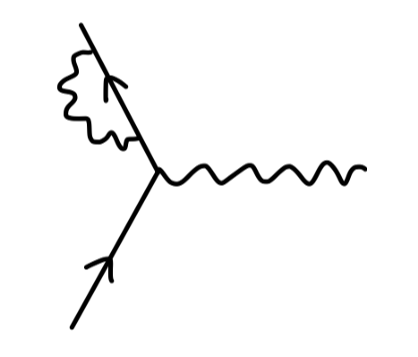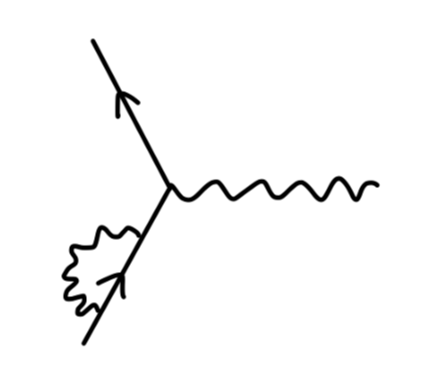• 真空极化 vacuum polarization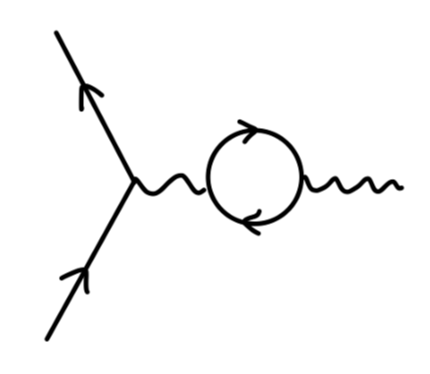• 顶点修正 vertex correction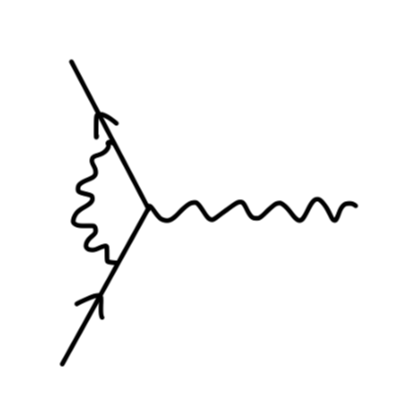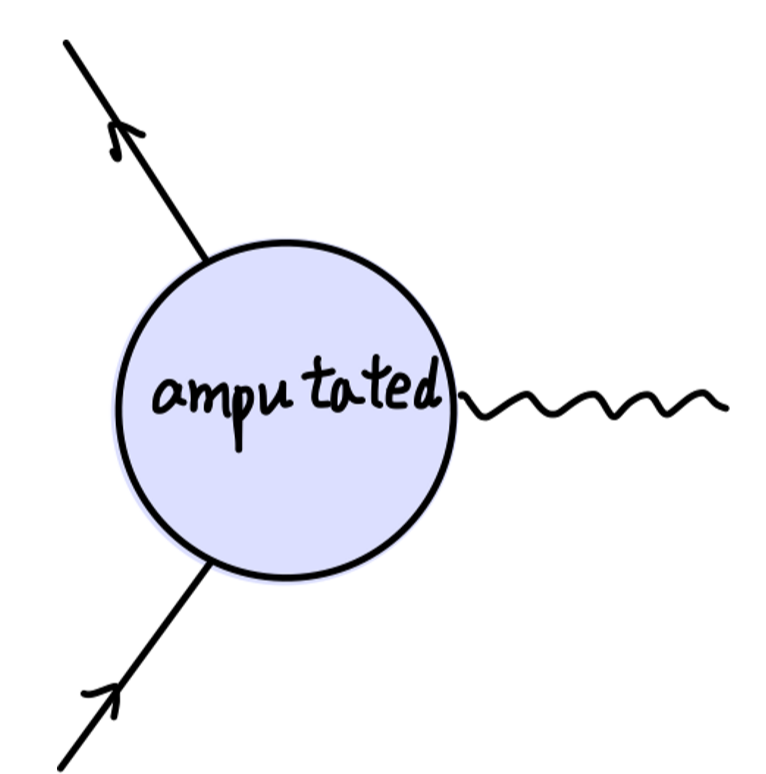$i\Gamma^{\mu}(p,p')$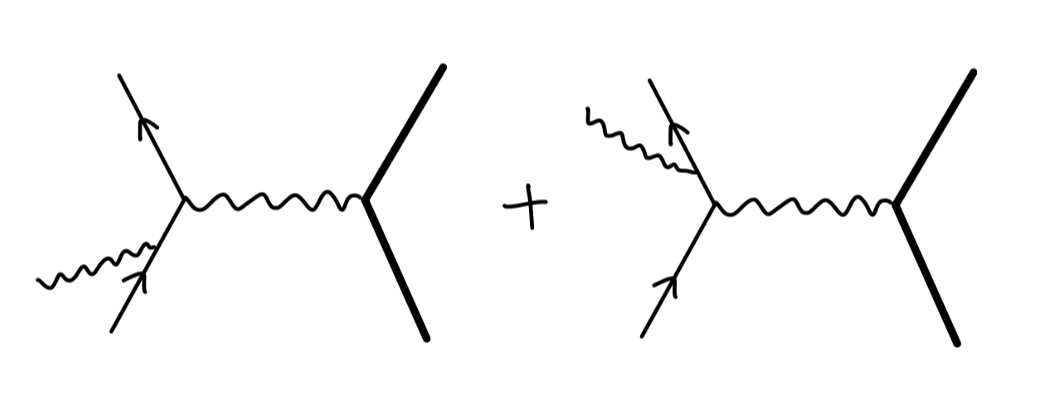## 轫致辐射

### 轫致辐射的经典计算

\begin{aligned} j^{\mu}(x) &= (1,\bm{0})^{\mu} \cdot e\delta^{(3)}(\bm{x})\\ &= \int dt (1,\bm{0})^{\mu}\cdot e\delta^{(4)}(x-y(t))\qquad y^{\mu}(t) = (t,\bm{0})^{\mu}\\ \end{aligned}\tag{1}

$j^{\mu}(x) = e\int d\tau \frac{dy^{\mu}(\tau)}{d\tau}\delta^{(4)}(x-y(\tau))\tag{2}$

y^{\mu}(\tau) = \left\{ \begin{aligned} &(p^{\mu}/m) \tau \quad &\tau<0\\ &(p'^{\mu}/m) \tau\quad &\tau>0\\ \end{aligned} \right.\tag{3}

$j^{\mu}(x) = e\int_{0}^{\infty}d\tau \frac{p'^{\mu}}{m}\delta^{(4)}(x-\frac{p'}{m}\tau) + e\int_{-\infty}^{0}d\tau \frac{p^{\mu}}{m}\delta^{(4)}(x-\frac{p}{m}\tau)\tag{4}$

\begin{aligned} \tilde{j}^{\mu}(k) &= \int d^4x e^{ik\cdot x}j^{\mu}(x)\\ &= e\int_{0}^{\infty} d\tau \frac{p'^{\mu}}{m} e^{i(kp'/m+i\epsilon)\tau} + e\int_{-\infty}^{0} d\tau \frac{p^{\mu}}{m} e^{i(kp/m-i\epsilon)\tau}\\ &= ie(\frac{p'^{\mu}}{k\cdot p' + i\epsilon}-\frac{p^{\mu}}{k\cdot p - i\epsilon}) \end{aligned}\tag{5}

$\partial^2 A^{\mu} = j^{\mu}$

$\tilde{A}^{\mu}(k) = -\frac{1}{k^2}\tilde{j}^{\mu}(k) \tag{6}$

$A^{\mu}(x) = \int \frac{d^4k}{(2\pi)^4}e^{-ik\cdot x}\frac{-ie}{k^2}(\frac{p'^{\mu}}{k\cdot p' + i\epsilon}-\frac{p^{\mu}}{k\cdot p - i\epsilon})\tag{7}$

\begin{aligned} &k^0 = -|\bm{k}|\\ &k^0 = +|\bm{k}|\\ &k \cdot p = 0\\ &k \cdot p' = 0\\ \end{aligned}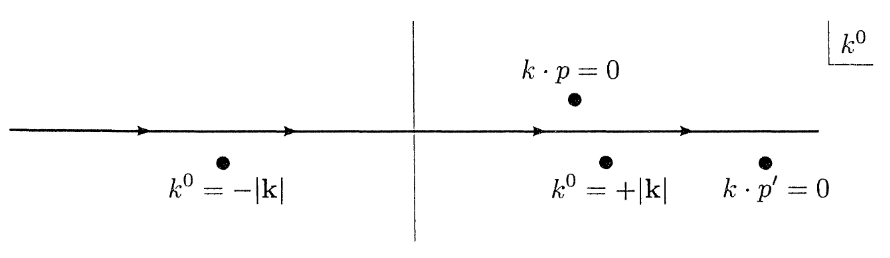$k^0 = \frac{\bm{k}\cdot\bm{p}}{p^0}$

\begin{aligned} A^{\mu}(x) &= \int \frac{d^3k}{(2\pi)^3} e^{i\bm{k}\cdot \bm{x}} e^{-i(\bm{k}\cdot\bm{p}/p^0)t} \frac{2\pi i (+ie)}{2\pi k^2} \frac{p^{\mu}}{p^0}\\ &= \int \frac{d^3k}{(2\pi)^3} e^{i\bm{k}\cdot \bm{x}} e^{-i(\bm{k}\cdot\bm{p}/p^0)t} \frac{-e}{k^2} \frac{p^{\mu}}{p^0}\\ \end{aligned}

$A^{\mu}(x) = \int \frac{d^3k}{(2\pi)^3} e^{i\bm{k}\cdot\bm{x}} \frac{e}{|\bm{k}|^2}\cdot(1,\bm{0})$

$t>0$ 时，我们选取围线向下闭合。此时围线内包含三个极点，其中极点 $k^0 = \bm{k}\cdot\bm{p}'/p'^{0}$ 表示出射粒子库伦势的贡献。我们重点关注剩下两个极点 $k^0 = \pm |\bm{k}|$ 的贡献，它们构成了辐射场：

\begin{aligned} A^{\mu}_{rad}(x) &= -\int \frac{d^3k}{(2\pi)^3} \frac{e}{2|\bm{k}|}\{ e^{-ik\cdot x}(\frac{p'^{\mu}}{k\cdot p'}-\frac{p^{\mu}}{k\cdot p}) + c.c \}|_{k^0 = |\bm{k}|}\\ &= \mathrm{Re} \int \frac{d^3k}{(2\pi)^3}\mathcal{A}^{\mu}(\bm{k})e^{-ik\cdot x} \end{aligned}\tag{8}

$\mathcal{A}^{\mu}(\bm{k}) = \frac{-e}{|\bm{k}|}(\frac{p'^{\mu}}{k\cdot p'}-\frac{p^{\mu}}{k\cdot p})\tag{9}$

\begin{aligned} \mathcal{E}(\bm{k}) &= -\bm{k}\mathcal{A}^0(\bm{k}) + ik^0 \mathcal{A}(\bm{k})\\ \mathcal{B}(\bm{k}) &= i\bm{k}\times\mathcal{A}(\bm{k})=\hat{k}\times\mathcal{E}(\bm{k})\\ \end{aligned}\tag{10}

\begin{aligned} \bm{E}(x) = \mathrm{Re}\int\frac{d^3k}{(2\pi)^3} \mathcal{E}(\bm{k})e^{-ik\cdot x}\\ \bm{B}(x) = \mathrm{Re}\int\frac{d^3k}{(2\pi)^3} \mathcal{B}(\bm{k})e^{-ik\cdot x}\\ \end{aligned}\tag{11}

\begin{aligned} E &= \frac{1}{2}\int d^3x (|\bm{E}(x)|^2 + |\bm{B}(x)|^2)\\ \end{aligned}\tag{12}

$(11)$ 式代入 $(12)$ 计算总能量，以及利用 $(10)$ 式，化简得到：

$E = \frac{1}{2}\int \frac{d^3k}{(2\pi)^3}\mathcal{E}(\bm{k})\cdot \mathcal{E}^*(\bm{k})\tag{13}$

$\mathcal{E}(\bm{k})\mathcal{E}^*(\bm{k}) = \sum_{\lambda=1,2}|\epsilon_{\lambda}(\bm{k})\cdot\mathcal{E}(\bm{k})|^2 = |\bm{k}|^2\sum_{\lambda=1,2}|\epsilon_{\lambda}(\bm{k})\cdot\mathcal{A}(\bm{k})|^2$

\begin{aligned} E &= \frac{1}{2}\int \frac{d^3k}{(2\pi)^3}\mathcal{E}(\bm{k})\cdot \mathcal{E}^*(\bm{k}) \\ &= \frac{1}{2}\int \frac{d^3k}{(2\pi)^3} |\bm{k}|^2\sum_{\lambda=1,2}|\bm{\epsilon}_{\lambda}(\bm{k})\cdot\mathcal{A}(\bm{k})|^2\\ &= \frac{1}{2}\int \frac{d^3k}{(2\pi)^3} e^2 \sum_{\lambda=1,2}|\bm{\epsilon}_{\lambda}(\bm{k})\cdot(\frac{\bm{p}'}{k\cdot p'}-\frac{\bm{p}}{k\cdot p})|^2\\ \end{aligned}

$E = \frac{1}{2}\int \frac{d^3k}{(2\pi)^3} e^2 \sum_{\lambda=1,2}|\epsilon_{\lambda}(\bm{k})\cdot(\frac{p'}{k\cdot p'}-\frac{p}{k\cdot p})|^2 \tag{14}$

$\mathcal{M}^{\mu}(k) = \frac{p'^{\mu}}{k\cdot p'}-\frac{p^{\mu}}{k\cdot p}$

$k_{\mu}\mathcal{M}^{\mu}(k) = 0$

$k\mathcal{M}^{0}(\bm{k}) - k\mathcal{M}^{3}(\bm{k}) = 0 \Rightarrow \mathcal{M}^{0}(\bm{k}) = \mathcal{M}^{3}(\bm{k})$

$\epsilon_1 = (0,1,0,0)\quad \epsilon_2 = (0,0,1,0)$

\begin{aligned} \sum_{\lambda=1,2}|\epsilon_{\lambda}(\bm{k})\cdot\mathcal{M}(\bm{k})|^2 &= \sum_{\lambda = 1,2} \epsilon_{\lambda\mu}(\bm{k})\epsilon_{\lambda\nu}^*(\bm{k})\mathcal{M}^{\mu}(\bm{k})\mathcal{M}^{\nu*}(\bm{k})\\ &= |\mathcal{M}^1(\bm{k})|^2 + |\mathcal{M}^2(\bm{k})|^2\\ &= -|\mathcal{M}^0(\bm{k})|^2 + |\mathcal{M}^1(\bm{k})|^2 + |\mathcal{M}^2(\bm{k})|^2 + |\mathcal{M}^3(\bm{k})|^2\\ & = -g_{\mu\nu}\mathcal{M}^\mu(\bm{k})\mathcal{M}^{\nu*}(\bm{k}) \end{aligned}

$\sum\epsilon_{\mu}\epsilon_{\nu}^{*} = -g_{\mu\nu}$

\begin{aligned} E &= \int \frac{d^3k}{(2\pi)^3} \frac{e^2}{2}(-g_{\mu\nu})(\frac{p'^{\mu}}{k\cdot p'}-\frac{p^{\mu}}{k\cdot p})(\frac{p'^{\nu}}{k\cdot p'}-\frac{p^{\nu}}{k\cdot p})\\ &= \int \frac{d^3k}{(2\pi)^3}\frac{e^2}{2}(-\frac{p'^2}{(k \cdot p')^2} + \frac{2p\cdot p'}{(k\cdot p)(k \cdot p')} -\frac{p^2}{(k \cdot p)^2} )\\ &= \int \frac{d^3k}{(2\pi)^3}\frac{e^2}{2}(-\frac{m^2}{(k \cdot p')^2} + \frac{2p\cdot p'}{(k\cdot p)(k \cdot p')} -\frac{m^2}{(k \cdot p)^2} )\\ &= \frac{e^2}{(2\pi)^2} \int dk \mathcal{I}(\bm{v},\bm{v}') \end{aligned}\tag{15}

$p^{\mu} = E(1,\bm{v})\quad p'^{\mu} = E(1,\bm{v}')\quad k^{\mu} = (k,\bm{k})$

$\mathcal{I}(\bm{v},\bm{v}') = \int \frac{d\Omega_{k}}{4\pi} (\frac{2(1-\bm{v}\cdot\bm{v}')}{(1-\hat{k}\cdot\bm{v})(1-\hat{k}\cdot\bm{v}')} - \frac{m^2/E^2}{(1-\hat{k}\cdot \bm{v}')^2}- \frac{m^2/E^2}{(1-\hat{k}\cdot \bm{v})^2})\tag{16}$

$E = \frac{\alpha}{\pi}k_{max}\mathcal{I}(\bm{v},\bm{v}')$

$\mathcal{I}(\bm{v},\bm{v}') \approx 2\log (-\frac{q^2}{m^2})$

$N = \frac{\alpha}{\pi} \int_{0}^{k_{max}} dk \frac{1}{k}\mathcal{I}(\bm{v},\bm{v}')$

### 轫致辐射的量子计算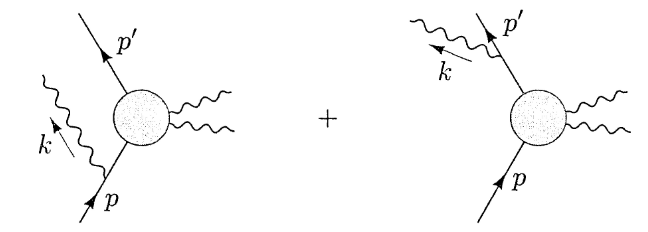\begin{aligned} i\mathcal{M} &= ie\bar{u}(p')(\mathcal{M}_0(p',p-k)\frac{i(\gamma\cdot(p-k)+m)}{(p-k)^2-m^2}\gamma^{\mu}\epsilon_{\mu}^*(k)\\ &\qquad +\gamma^{\mu}\epsilon_{\mu}^*(k)\frac{i(\gamma\cdot(p'+k)+m)}{(p'+k)^2-m^2}\mathcal{M}_0(p'+k,p))u(p)\\ & \approx \bar{u}(p')(M_0(p',p))u(p)\cdot(e(\frac{p'\cdot \epsilon^*}{p'\cdot k}-\frac{p\cdot \epsilon^*}{p\cdot k})) \end{aligned}\tag{17}

$\mathcal{M}_0(p',p-k) \approx \mathcal{M}_0(p'+k,p) \approx \mathcal{M}_0(p',p)$

\begin{aligned} (\gamma\cdot p + m)\gamma^{\mu}\epsilon_{\mu}^* u(p) &= (2p^{\mu}\epsilon_{\mu}^*+\gamma^{\mu}\epsilon_{\mu}^*(-\gamma\cdot p + m))u(p)\\ &=2p^{\mu}\epsilon_{\mu}^* u(p)\\ \end{aligned}

\begin{aligned} d\sigma(p\rightarrow p' + \gamma) &= d\sigma(p\rightarrow p')\cdot \int \frac{d^3k}{(2\pi)^3}\frac{1}{2k}\sum_{\lambda=1,2} e^2|\frac{p'\cdot \epsilon^{(\lambda)}}{p'\cdot k}-\frac{p\cdot \epsilon^{(\lambda)}}{p\cdot k}|^2\\ \end{aligned}\tag{18}

$d(prob) = \frac{d^3k}{(2\pi)^3}\sum_{\lambda=1,2} \frac{e^2}{2k}|\frac{p'\cdot \epsilon^{(\lambda)}}{p'\cdot k}-\frac{p\cdot \epsilon^{(\lambda)}}{p\cdot k}|^2$

$\frac{\alpha}{\pi}\int_{0}^{|\bm{q}|}dk \frac{1}{k}\mathcal{I}(\bm{v},\bm{v}')$

\begin{aligned} d\sigma(p\rightarrow p' + \gamma) &= d\sigma(p\rightarrow p')\cdot \frac{\alpha}{2\pi} \log(-\frac{q^2}{\mu^2}) \mathcal{I}(\bm{v},\bm{v}')\\ &\overset{-q^2\rightarrow\infty}{\approx}d\sigma(p\rightarrow p')\cdot \frac{\alpha}{\pi} \log(-\frac{q^2}{\mu^2}) \log (-\frac{q^2}{m^2}) \end{aligned} \tag{19}